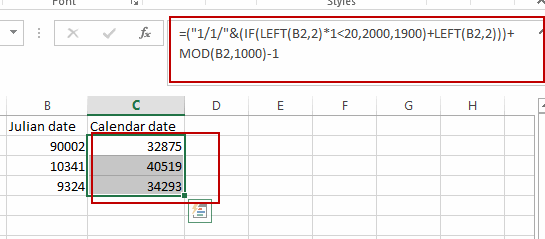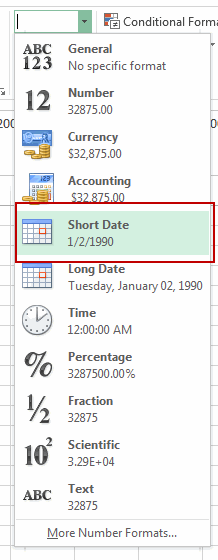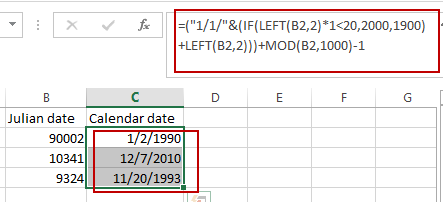# How to Convert Julian Date to a Calendar date in Excel

This post will guide you how to convert Julian date to a calendar date in Excel. How do I convert a given Julian Date to a calendar date with a formula in Excel.

## What is Julian Date?

Julian day number  is the integer assigned to a whole solar day in the Julian day count starting from noon Universal time, with Julian day number 0 assigned to the day starting at noon on Monday, January 1, 4713 BC, proleptic Julian calendar (November 24, 4714 BC, in the proleptic Gregorian calendar), a date at which three multi-year cycles started (which are: Indiction, Solar, and Lunar cycles) and which preceded any dates in recorded history.

## Convert Julian Date to Calendar date

If you want to convert Julian date to a calendar date for your date values, and you can use a formula based on the IF function, the LEFT function and the MOD function. Like this:

`=("1/1/"&(IF(LEFT(B2,2)*1<20,2000,1900)+LEFT(B2,2)))+MOD(B2,1000)-1`

Type this formula into cell c2, and press Enter key on your keyboard to apply this formula.Then go to HOME tab, select Date as Number Format in the Number group.Keep to select the Cell C2, and drag the AutoFill Handle down to other cells to apply this formula to convert the Julian date format into Calendar date.### Related Functions

• Excel IF function
The Excel IF function perform a logical test to return one value if the condition is TRUE and return another value if the condition is FALSE. The IF function is a build-in function in Microsoft Excel and it is categorized as a Logical Function.The syntax of the IF function is as below:= IF (condition, [true_value], [false_value])….
• Excel MOD function
he Excel MOD function returns the remainder of two numbers after division. So you can use the MOD function to get the remainder after a number is divided by a divisor in Excel. The syntax of the MOD function is as below:=MOD (number, divisor)….
• Excel LEFT function
The Excel LEFT function returns a substring (a specified number of the characters) from a text string, starting from the leftmost character.The LEFT function is a build-in function in Microsoft Excel and it is categorized as a Text Function.The syntax of the LEFT function is as below:= LEFT(text,[num_chars])…t)…

### You might also like:

Sidebar